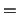Next: Tutorials and Examples Up: User-Defined Graphics Previous: User-Defined Graphics   Contents   Index

Introduction

There are 10 types of basic graphical items built into VMD, which are used with graphics and draw commands. These are:

• point {x y z} -

draws a point at the given position

• line {x1 y1 z1} {x2 y2 z2} [width w] [stylesolid|dashed] -

draws either a solid or dashed line of the given width from the first point to the second. By default, this is a solid line of width 1.

• cylinder {x1 y1 z1} {x2 y2 z2} [radius r] [resolution n] [filledyes|no] -

draws a cylinder of the given radius (default r1) from the first point to the second. The cylinder is actually drawn as an n sided polygon. If the filled option is true (default), the ends are capped with flat disks, otherwise the cylinder is hollow.

• cone {basex basey basez} {tipz tipy tipz} [radius r] [resolution n] -

draw a cone with the center of the base at the first point and the tip at the second. The radius(default r1) determines the width of the base. As with cylinder, the resolution determines the number of polygons used in the approximation.

• triangle {x1 y1 z1} {x2 y2 z3} {x3 y3 z3} -

draws a triangle with endpoints at each of the three vertices

• trinorm {x1 y1 z1} {x2 y2 z3} {x3 y3 z3} {nx1 y1 z1} {nx2 ny2 nz3} {nx3 ny3 nz3} -

draws a triangle with endpoints at each of the first three points. The second group of three values specify the normals for the three points. This is used for making a smooth shading across the triangle.

• sphere {x y z} [radius r] [resolution n] -

draws a sphere of the given radius (default r1) centered at the vertex. The resolution (default n6) determines how many polygons are used in the approximation of a sphere.

• text {x y z} ``text string'' -

displays the text string with the bottom left of the string starting at the given vertex.

• color colorId -
• color name -
• color trans_name - Each of the above geometrical objects are drawn using the current color. Initially, that color is blue, which has the colorid of 0. The color command changes the current color, and stays info effect until the next color command. Thus, to draw a red cylinder then a red sphere, first use the command color red command to change the color, then use the cylinder and sphere commands.

• materialson|off- Material properties are used to make the graphical objects (lines, cylinders, etc.) be affected by the light sources. These make the objects look more realistic, but are slower on machines which don't implement materials in hardware (see chapter §  and sections on color and colorinfo commands for the information on how to turn off material characteristics for all objects in VMD). One surprising effect of material characteristics is that lines are affected. In some lighting situations, the lines can even appear to disappear. Thus, you may want to turn off materials before drawing lines.

• materialname- Sets the material to use for the corresponding graphics molecule. name must be a valid material name, as displayed in the Materials menu.Next: Tutorials and Examples Up: User-Defined Graphics Previous: User-Defined Graphics   Contents   Index
vmd@ks.uiuc.edu